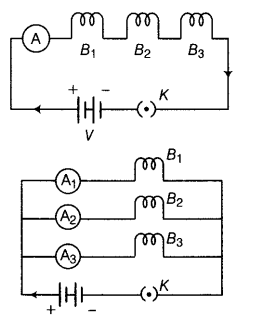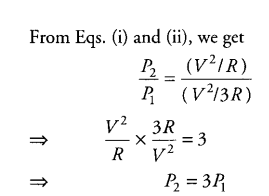# Three incandescent bulbs of 100 W each are connected in series in an electric circuit

Three incandescent bulbs of 100 W each are connected in series in an electric circuit. In another set of three bulbs of the same wattage are connected in parallel to the source.
(i) Will the bulb in the two circuits glow with the same brightness? Justify your answer.
(ii) Now, let one bulb in both the circuits get fused. Will the rest of the bulbs continue to glow in each circuit? Give reason.

(i) Let us assume that the resistance of each bulb be R.
The circuit diagram in two cases may be drawn as given below:Therefore, each bulb in parallel combination glows 3 times brighter to that of each bulb in series combination.
(ii) When one bulb gets fused in both the circuits, then in series combination, circuit gets broken and current stops flowing, whereas in parallel combination, same voltage continues to act on the remaining bulbs and hence other bulbs continues to glow with same brightness.

Everything is right except the fact that you have taken R as 3R of each bulb and arrived at P2 = 3P1. It should be P2 = 9P1.

Let the source voltage be V’

Hence, I = V’/3R
Thus, power through each bulb = I^2R = V’^2/9R
In Parallel, bulbs are connected to the same source i.e. voltage = V’

Therefore, power through each bulb = V’^2/R.
Do the math.
CheersWhen the Power of each bulb has already been specified, how can we then compare them?

I am a little confused here!